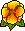## Mellin analysis of weighted Sobolev spaces with nonhomogeneous norms on cones

Martin Costabel, Monique Dauge, Serge NicaiseOn domains with conical points, weighted Sobolev spaces with powers of the distance to the conical points as weights form a classical framework for describing the regularity of solutions of elliptic boundary value problems, cf. papers by Kondrat'ev and Maz'ya-Plamenevskii. Two classes of weighted norms are usually considered: Homogeneous norms, where the weight exponent varies with the order of derivatives, and nonhomogeneous norms, where the same weight is used for all orders of derivatives. For the analysis of the spaces with homogeneous norms, Mellin transformation is a classical tool.

In this paper, we show how Mellin transformation can also be used to give an optimal characterization of the structure of weighted Sobolev spaces with nonhomogeneous norms on finite cones in the case of both non-critical and critical indices. This characterization can serve as a basis for the proof of regularity and Fredholm theorems in such weighted Sobolev spaces on domains with conical points, even in the case of critical indices.In Around the Research of Vladimir Maz'ya I, Function Spaces.
International Mathematical Series (Springer) Vol. 11, pp 105-136 (2010).Fichier pdf (360 k)